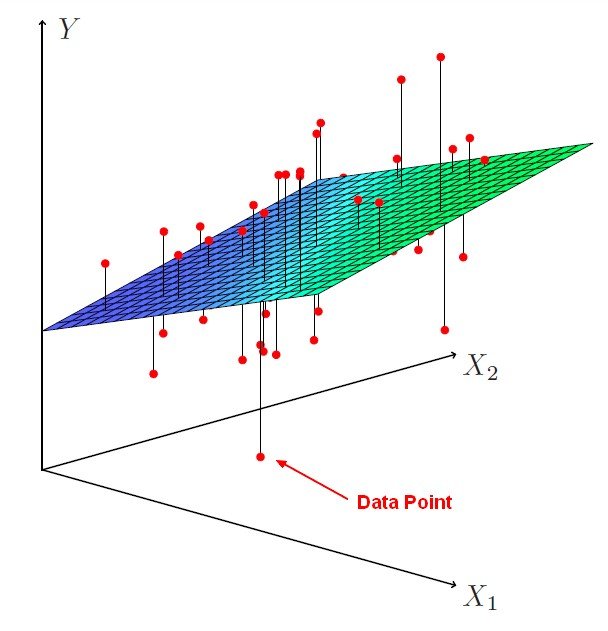# Statistics - F-distributions

Like the t-test and family of t-distributions, the F-test has a family of F-distributions

## F-distributions

The family of F-distributions depends on:

• the Number of subjects per group
• the Number of groups

ANOVA can handle as many groups as you like. So there are different sampling distributions. This different sampling distributions can be generated in R.

Each different curve varies depending on the number of groups and number of people in a group.

It's not symmetrical because negative values are impossible. It's a ratio of variances. The expected value under the null hypothesis is just one.

It's the same concept as t and z distributions but it just looks a little different.

Recommended PagesStatistics - (F-Statistic|F-test|F-ratio)

The NHST anova statistic test is an F-test or F-ratio. It's what you observe in the numerator relative to what you would expect just due to chance in the denominator. The f statistic is the statistic...Statistics - Multiple Linear Regression

Multiple regression is a regression with multiple predictors. It extends the simple model. You can have many predictor as you want. The power of multiple regression (with multiple predictor) is to better...